login  home  contents  what's new  discussion  bug reports help  links  subscribe  changes  refresh  edit

The Pauli Algebra Cl(3) Is Frobenius In Many Ways

Linear operators over a 8-dimensional vector space representing Pauli algebra

Ref:

We need the Axiom LinearOperator library.

fricas
)library CARTEN ARITY CMONAL CPROP CLOP CALEY
CartesianTensor is now explicitly exposed in frame initial
CartesianTensor will be automatically loaded when needed from
/var/aw/var/LatexWiki/CARTEN.NRLIB/CARTEN
Arity is now explicitly exposed in frame initial
Arity will be automatically loaded when needed from
/var/aw/var/LatexWiki/ARITY.NRLIB/ARITY
ClosedMonoidal is now explicitly exposed in frame initial
ClosedMonoidal will be automatically loaded when needed from
/var/aw/var/LatexWiki/CMONAL.NRLIB/CMONAL
ClosedProp is now explicitly exposed in frame initial
ClosedProp will be automatically loaded when needed from
/var/aw/var/LatexWiki/CPROP.NRLIB/CPROP
ClosedLinearOperator is now explicitly exposed in frame initial
ClosedLinearOperator will be automatically loaded when needed from
/var/aw/var/LatexWiki/CLOP.NRLIB/CLOP
CaleyDickson is now explicitly exposed in frame initial
CaleyDickson will be automatically loaded when needed from
/var/aw/var/LatexWiki/CALEY.NRLIB/CALEY

Use the following macros for convenient notation

fricas
-- summation
macro Σ(x,i,n)==reduce(+,[x for i in n])
Type: Void
fricas
-- list
macro Ξ(f,i,n)==[f for i in n]
Type: Void
fricas
-- subscript and superscripts
macro sb == subscript
Type: Void
fricas
macro sp == superscript
Type: Void

𝐋 is the domain of 8-dimensional linear operators over the rational functions ℚ (Expression Integer), i.e. ratio of polynomials with integer coefficients.

fricas
dim:=8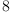(1)
Type: PositiveInteger?
fricas
macro ℒ == List
Type: Void
fricas
macro ℂ == CaleyDickson
Type: Void
fricas
macro ℚ == Expression Integer
Type: Void
fricas
𝐋 := ClosedLinearOperator(OVAR ['1,'i,'j,'k,'ij,'ik,'jk,'ijk], ℚ)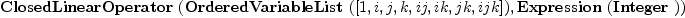(2)
Type: Type
fricas
𝐞:ℒ 𝐋      := basisOut()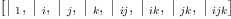(3)
Type: List(ClosedLinearOperator(OrderedVariableList([1,i,j,k,ij,ik,jk,ijk]),Expression(Integer)))
fricas
𝐝:ℒ 𝐋      := basisIn()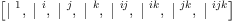(4)
Type: List(ClosedLinearOperator(OrderedVariableList([1,i,j,k,ij,ik,jk,ijk]),Expression(Integer)))
fricas
I:𝐋:=   -- identity for composition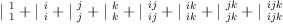(5)
Type: ClosedLinearOperator(OrderedVariableList([1,i,j,k,ij,ik,jk,ijk]),Expression(Integer))
fricas
X:𝐋:=[2,1] -- twist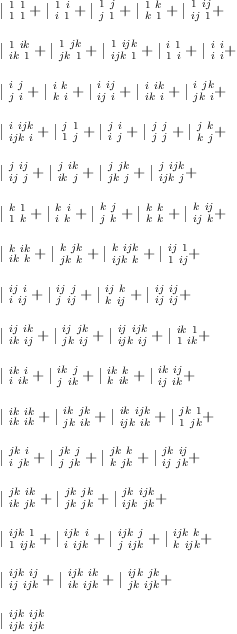(6)
Type: ClosedLinearOperator(OrderedVariableList([1,i,j,k,ij,ik,jk,ijk]),Expression(Integer))
fricas
V:𝐋:=ev(1) -- evaluation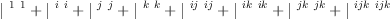(7)
Type: ClosedLinearOperator(OrderedVariableList([1,i,j,k,ij,ik,jk,ijk]),Expression(Integer))
fricas
Λ:𝐋:=co(1) -- co-evaluation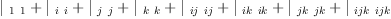(8)
Type: ClosedLinearOperator(OrderedVariableList([1,i,j,k,ij,ik,jk,ijk]),Expression(Integer))
fricas
equate(eq)==map((x,y)+->(x=y),ravel lhs eq, ravel rhs eq);
Type: Void

Now generate structure constants for Pauli Algebra

The basis consists of the real and imaginary units. We use quaternion multiplication to form the "multiplication table" as a matrix. Then the structure constants can be obtained by dividing each matrix entry by the list of basis vectors.

The Pauli Algebra as Cl(3)

Basis: Each B.i is a Clifford number

fricas
q0:=sp('i,)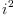(9)
Type: Symbol
fricas
q1:=sp('j,)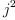(10)
Type: Symbol
fricas
q2:=sp('k,)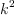(11)
Type: Symbol
fricas
QQ:=CliffordAlgebra(3,ℚ,matrix [[q0,0,0],[0,q1,0],[0,0,q2]])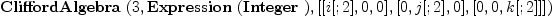(12)
Type: Type
fricas
B:ℒ QQ := [monomial(1,[]),monomial(1,),monomial(1,),monomial(1,),monomial(1,[1,2]),monomial(1,[1,3]),monomial(1,[2,3]),monomial(1,[1,2,3])]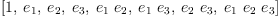(13)
Type: List(CliffordAlgebra?(3,Expression(Integer),[[i[;2],0,0],[0,j[;2],0],[0,0,k[;2]]]))
fricas
M:Matrix QQ := matrix Ξ(Ξ(B.i*B.j, i,1..dim), j,1..dim)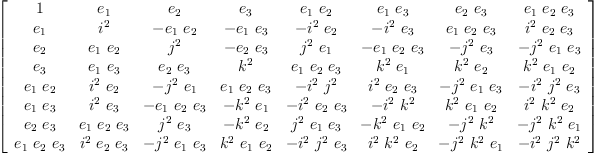(14)
Type: Matrix(CliffordAlgebra?(3,Expression(Integer),[[i[;2],0,0],[0,j[;2],0],[0,0,k[;2]]]))
fricas
S(y) == map(x +-> coefficient(recip(y)*x,[]),M)
Type: Void
fricas
ѕ :=map(S,B)::ℒ ℒ ℒ ℚ
fricas
Compiling function S with type CliffordAlgebra(3,Expression(Integer)
,[[i[;2],0,0],[0,j[;2],0],[0,0,k[;2]]]) -> Matrix(Expression(
Integer))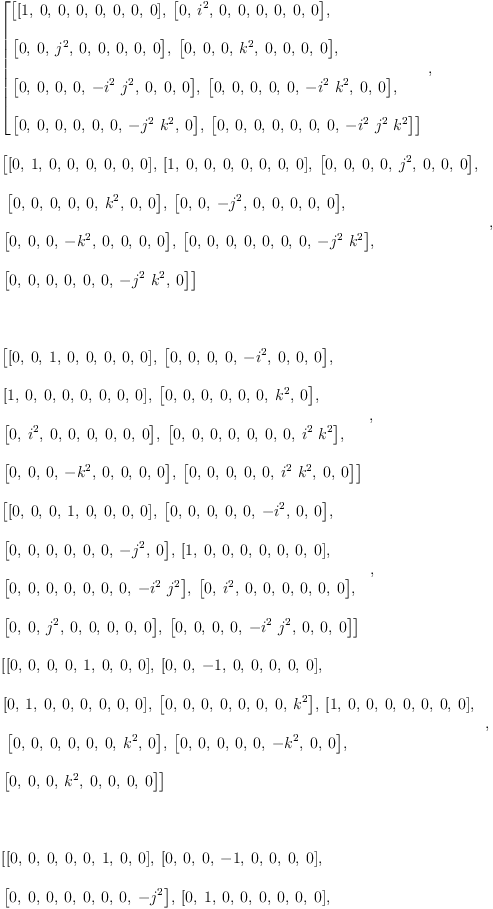(15)
Type: List(List(List(Expression(Integer))))
fricas
-- structure constants form a tensor operator
Y := Σ(Σ(Σ(ѕ(i)(k)(j)*𝐞.i*𝐝.j*𝐝.k, i,1..dim), j,1..dim), k,1..dim)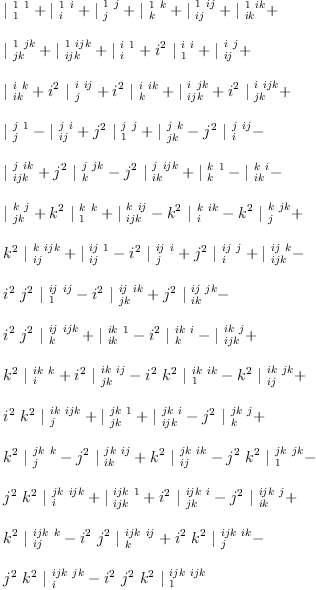(16)
Type: ClosedLinearOperator(OrderedVariableList([1,i,j,k,ij,ik,jk,ijk]),Expression(Integer))
fricas
matrix Ξ(Ξ((𝐞.i*𝐞.j)/Y, i,1..dim), j,1..dim)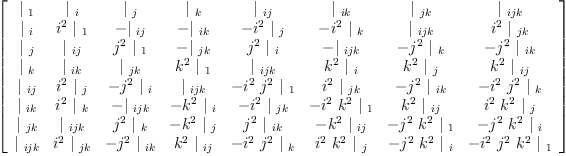(17)
Type: Matrix(ClosedLinearOperator(OrderedVariableList([1,i,j,k,ij,ik,jk,ijk]),Expression(Integer)))
fricas
XY := X/Y;
Type: ClosedLinearOperator(OrderedVariableList([1,i,j,k,ij,ik,jk,ijk]),Expression(Integer))

Units

fricas
e:=𝐞.1; i:=𝐞.2; j:=𝐞.3; k:=𝐞.4; ij:=𝐞.5; ik:=𝐞.6; jk:=𝐞.7; ijk:=𝐞.8;
Type: ClosedLinearOperator(OrderedVariableList([1,i,j,k,ij,ik,jk,ijk]),Expression(Integer))

Multiplication of arbitrary quaternions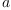and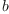fricas
a:=Σ(sb('a,[i])*𝐞.i, i,1..dim)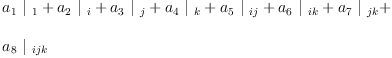(18)
Type: ClosedLinearOperator(OrderedVariableList([1,i,j,k,ij,ik,jk,ijk]),Expression(Integer))
fricas
b:=Σ(sb('b,[i])*𝐞.i, i,1..dim)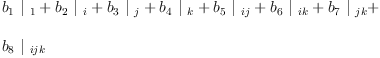(19)
Type: ClosedLinearOperator(OrderedVariableList([1,i,j,k,ij,ik,jk,ijk]),Expression(Integer))
fricas
(a*b)/Y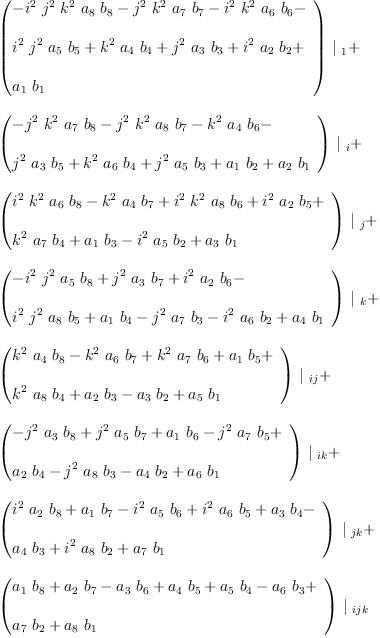(20)
Type: ClosedLinearOperator(OrderedVariableList([1,i,j,k,ij,ik,jk,ijk]),Expression(Integer))

Multiplication is Associative

fricas
test(
( I Y ) / _
(  Y  ) = _
( Y I ) / _
(  Y  ) )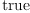(21)
Type: Boolean

A scalar product is denoted by the (2,0)-tensor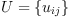fricas
U:=Σ(Σ(script('u,[[],[i,j]])*𝐝.i*𝐝.j, i,1..dim), j,1..dim)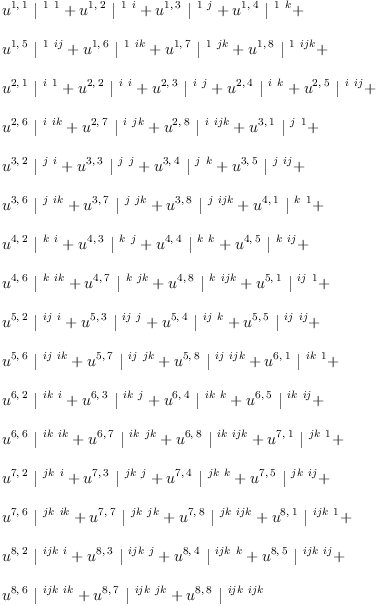(22)
Type: ClosedLinearOperator(OrderedVariableList([1,i,j,k,ij,ik,jk,ijk]),Expression(Integer))

## Definition 1

We say that the scalar product is associative if the tensor equation holds:

    Y   =   Y
U     U


In other words, if the (3,0)-tensor: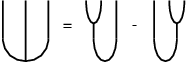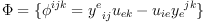(23)
(three-point function) is zero.

Using the LinearOperator domain in Axiom and some carefully chosen symbols we can easily enter expressions that are both readable and interpreted by Axiom as "graphical calculus" diagrams describing complex products and compositions of linear operators.

fricas
ω:𝐋 :=                 _
(    Y I    )  /  _
U        -  _
(    I Y    )  /  _
U;
Type: ClosedLinearOperator(OrderedVariableList([1,i,j,k,ij,ik,jk,ijk]),Expression(Integer))

## Definition 2

An algebra with a non-degenerate associative scalar product is called a [Frobenius Algebra]?.

The Cartan-Killing Trace

fricas
Ú:=
(  Y Λ  ) / _
(   Y I ) / _
V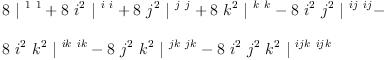(24)
Type: ClosedLinearOperator(OrderedVariableList([1,i,j,k,ij,ik,jk,ijk]),Expression(Integer))
fricas
Ù:=
(  Λ Y  ) / _
( I Y   ) / _
V(25)
Type: ClosedLinearOperator(OrderedVariableList([1,i,j,k,ij,ik,jk,ijk]),Expression(Integer))
fricas
test(Ù=Ú)(26)
Type: Boolean

forms a non-degenerate associative scalar product for Y

fricas
Ũ := Ù(27)
Type: ClosedLinearOperator(OrderedVariableList([1,i,j,k,ij,ik,jk,ijk]),Expression(Integer))
fricas
test
(    Y I    )  /
Ũ        =
(    I Y    )  /
Ũ(28)
Type: Boolean
fricas
determinant Ξ(Ξ(retract((𝐞.i * 𝐞.j)/Ũ), j,1..dim), i,1..dim)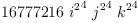(29)
Type: Expression(Integer)

General Solution

Frobenius Form (co-unit)

fricas
d:=ε1*𝐝.1+εi*𝐝.2+εj*𝐝.3+εk*𝐝.4+εij*𝐝.5+εik*𝐝.6+εjk*𝐝.7+εijk*𝐝.8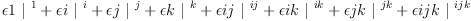(30)
Type: ClosedLinearOperator(OrderedVariableList([1,i,j,k,ij,ik,jk,ijk]),Expression(Integer))
fricas
Ų:= Y/d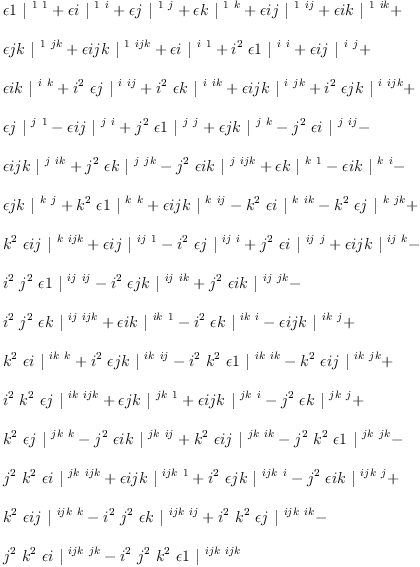(31)
Type: ClosedLinearOperator(OrderedVariableList([1,i,j,k,ij,ik,jk,ijk]),Expression(Integer))

In general the pairing is not symmetric!

fricas
u1:=matrix Ξ(Ξ(retract((𝐞.i 𝐞.j)/Ų), i,1..dim), j,1..dim)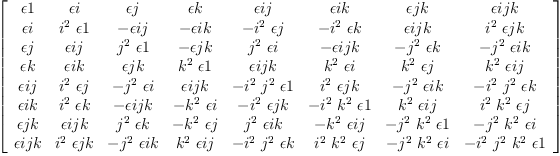(32)
Type: Matrix(Expression(Integer))

The scalar product must be non-degenerate:

fricas
--Ů:=determinant u1
--factor(numer Ů)/factor(denom Ů)
1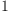(33)
Type: PositiveInteger?

Cartan-Killing is a special case

fricas
ck:=solve(equate(Ũ=Ų),[ε1,εi,εj,εk,εij,εik,εjk,εijk]).1
fricas
Compiling function equate with type Equation(ClosedLinearOperator(
OrderedVariableList([1,i,j,k,ij,ik,jk,ijk]),Expression(Integer)))
-> List(Equation(Expression(Integer)))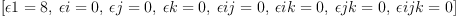(34)
Type: List(Equation(Expression(Integer)))

Frobenius scalar product of "vector" quaternionsandfricas
a:=sb('a,)*i+sb('a,)*j+sb('a,)*k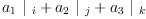(35)
Type: ClosedLinearOperator(OrderedVariableList([1,i,j,k,ij,ik,jk,ijk]),Expression(Integer))
fricas
b:=sb('b,)*i+sb('b,)*j+sb('b,)*k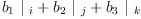(36)
Type: ClosedLinearOperator(OrderedVariableList([1,i,j,k,ij,ik,jk,ijk]),Expression(Integer))
fricas
(a,a)/Ų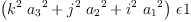(37)
Type: ClosedLinearOperator(OrderedVariableList([1,i,j,k,ij,ik,jk,ijk]),Expression(Integer))
fricas
(b,b)/Ų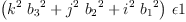(38)
Type: ClosedLinearOperator(OrderedVariableList([1,i,j,k,ij,ik,jk,ijk]),Expression(Integer))
fricas
(a,b)/Ų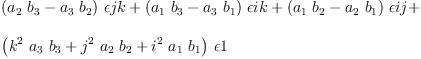(39)
Type: ClosedLinearOperator(OrderedVariableList([1,i,j,k,ij,ik,jk,ijk]),Expression(Integer))

## Definition 3

Co-scalar product

Solve the Snake Relation as a system of linear equations.

fricas
mU:=inverse matrix Ξ(Ξ(retract((𝐞.i*𝐞.j)/Ų), i,1..dim), j,1..dim);
Type: Union(Matrix(Expression(Integer)),...)
fricas
Ω:=Σ(Σ(mU(i,j)*(𝐞.i*𝐞.j), i,1..dim), j,1..dim);
Type: ClosedLinearOperator(OrderedVariableList([1,i,j,k,ij,ik,jk,ijk]),Expression(Integer))
fricas
ΩX:=Ω/X;
Type: ClosedLinearOperator(OrderedVariableList([1,i,j,k,ij,ik,jk,ijk]),Expression(Integer))

The common demoninator is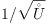fricas
--squareFreePart factor denom Ů / squareFreePart factor numer Ů
matrix Ξ(Ξ(numer retract(Ω/(𝐝.i*𝐝.j)), i,1..dim), j,1..dim)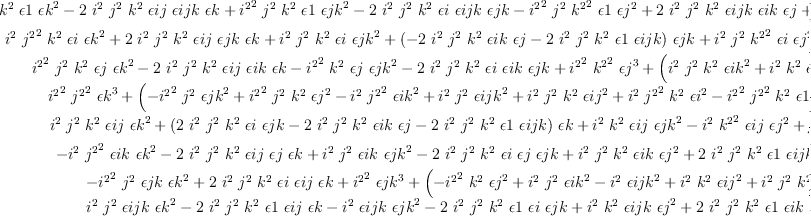(40)
Type: Matrix(SparseMultivariatePolynomial?(Integer,Kernel(Expression(Integer))))

Check "dimension" and the snake relations.

fricas
O:𝐋:= Ω / Ų(41)
Type: ClosedLinearOperator(OrderedVariableList([1,i,j,k,ij,ik,jk,ijk]),Expression(Integer))
fricas
test
(    I ΩX     )  /
(     Ų I     )  =  I(42)
Type: Boolean
fricas
test
(     ΩX I    )  /
(    I Ų      )  =  I(43)
Type: Boolean

Cartan-Killing co-scalar

fricas
eval(Ω,ck)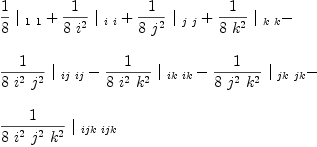(44)
Type: ClosedLinearOperator(OrderedVariableList([1,i,j,k,ij,ik,jk,ijk]),Expression(Integer))

## Definition 4

Co-algebra

Compute the "three-point" function and use it to define co-multiplication.

fricas
W:= (Y I) / Ų;
Type: ClosedLinearOperator(OrderedVariableList([1,i,j,k,ij,ik,jk,ijk]),Expression(Integer))

fricas
λ:=                      _
(    I ΩX     )  /  _
(     Y I     );
Type: ClosedLinearOperator(OrderedVariableList([1,i,j,k,ij,ik,jk,ijk]),Expression(Integer))
fricas
test
(     ΩX I    )  /
(    I  Y     )  =  λ(45)
Type: Boolean

Cartan-Killing co-multiplication

fricas
eval(λ,ck)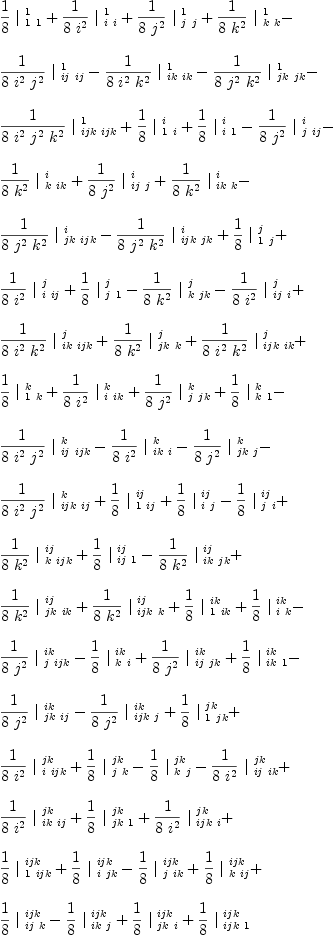(46)
Type: ClosedLinearOperator(OrderedVariableList([1,i,j,k,ij,ik,jk,ijk]),Expression(Integer))

fricas
test
e     /
λ     =    ΩX(47)
Type: Boolean

 Subject:   Be Bold !! ( 14 subscribers )